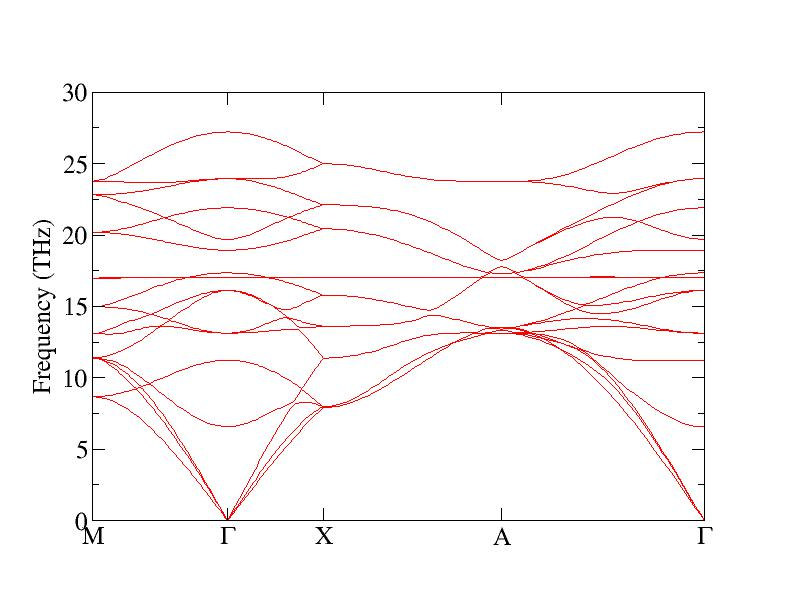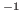Next: Density of states and Up: Description of the program Previous: Super-cell generation

## Phonon dispersions

To calculate the dispersions we need to add the following settings in the INPHON file:LRECIP = .T.
ND = 4; NPOINTS = 50
QI = 0.5 0.5 0.0   0.0 0.0 0.0   0.5 0.0 0.0   0.5 0.5 0.5

QF = 0.0 0.0 0.0   0.5 0.0 0.0   0.5 0.5 0.5   0.0 0.0 0.0

MASS = 28.085  16.0.

LRECIP = .T. means that the coordinates of the initial (QI) and final (QF) q-points are in units of reciprocal space lattice vectors. Set LRECIP=.F. if you want to give these numbers in Cartesian coordinates. ND is the number of initial and final points (usually high symmetry points in the Brillouin zone (BZ)), and NPOINTS is the number of points between them. MASS is the mass of the ions. We also need to add:

LSUPER= .F.

which will tell PHON to go beyond the generation of the super-cell (and not generating one). These settings will make PHON generate the file FREQ containing the frequencies in THz, and the file FREQ.cm with the frequencies in cm. If the number of atoms in the primitive cell is larger than 16 then FREQ and FREQ.cm are not written, and PHON produces a number of files FREQ1 .... FREQn, ... containing 48 phonon modes each (in THz units). By setting the variable:

LFORCEOUT=.TRUE. (default = .FALSE.)

The program writes the file HARMONIC which contains the force constant matrix.Next: Density of states and Up: Description of the program Previous: Super-cell generation
Dario Alfe` 2012-02-20